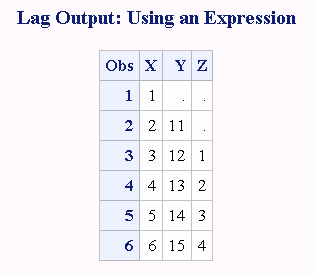# LAG Function

Returns values from a queue.

 Category: Special

## Syntax

LAG<n> (argument)

### Required Argument

#### argument

specifies a numeric or character constant, variable, or expression.

### Optional Argument

#### n

specifies the number of lagged values.

## Details

### The Basics

If the LAG function returns a value to a character variable that has not yet been assigned a length, by default the variable is assigned a length of 200.
The LAG functions, LAG1, LAG2, ..., LAGn return values from a queue. LAG1 can also be written as LAG. A LAGn function stores a value in a queue and returns a value stored previously in that queue. Each occurrence of a LAGn function in a program generates its own queue of values.
The queue for each occurrence of LAGn is initialized with n missing values, where n is the length of the queue (for example, a LAG2 queue is initialized with two missing values). When an occurrence of LAGn is executed, the value at the top of its queue is removed and returned, the remaining values are shifted upwards, and the new value of the argument is placed at the bottom of the queue. Hence, missing values are returned for the first n executions of each occurrence of LAGn, after which the lagged values of the argument begin to appear.
Note: Storing values at the bottom of the queue and returning values from the top of the queue occurs only when the function is executed. An occurrence of the LAGn function that is executed conditionally will store and return values only from the observations for which the condition is satisfied.
If the argument of LAGn is an array name, a separate queue is maintained for each variable in the array.

### Memory Limit for the LAG Function

When the LAG function is compiled, SAS allocates memory in a queue to hold the values of the variable that is listed in the LAG function. For example, if the variable in function LAG100(x) is numeric with a length of 8 bytes, then the memory that is needed is 8 times 100, or 800 bytes. Therefore, the memory limit for the LAG function is based on the memory that SAS allocates, which varies with different operating environments.

## Examples

### Example 1: Generating Two Lagged Values

The following program generates two lagged values for each observation.
```data one;
input x @@;
y=lag1(x);
z=lag2(x);
datalines;
1 2 3 4 5 6
;
proc print data=one;
title 'LAG Output';
run;```
Output from Generating Two Lagged Values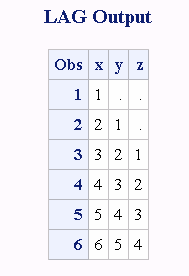LAG1 returns one missing value and the values of X (lagged once). LAG2 returns two missing values and the values of X (lagged twice).

### Example 2: Generating Multiple Lagged Values in BY-Groups

The following example shows how to generate up to three lagged values within each BY group.
```/***************************************************************************/
/* This program generates up to three lagged values.  By increasing the    */
/* size of the array and the number of assignment statements that use      */
/* the LAGn functions, you can generate as many lagged values as needed.   */
/***************************************************************************/
/* Create starting data. */
data old;
input start end;
datalines;
1 1
1 2
1 3
1 4
1 5
1 6
1 7
2 1
2 2
3 1
3 2
3 3
3 4
3 5
;
data new(drop=i count);
set old;
by start;
/* Create and assign values to three new variables.  Use ENDLAG1-      */
/* ENDLAG3 to store lagged values of END, from the most recent to the  */
/* third preceding value.                                              */
array x(*) endlag1-endlag3;
endlag1=lag1(end);
endlag2=lag2(end);
endlag3=lag3(end);
/* Reset COUNT at the start of each new BY-Group */
if first.start then count=1;
/* On each iteration, set to missing array elements   */
/* that have not yet received a lagged value for the  */
/* current BY-Group.  Increase count by 1.            */
do i=count to dim(x);
x(i)=.;
end;
count + 1;
run;
proc print;
run;
```
Output from Generating Three Lagged Values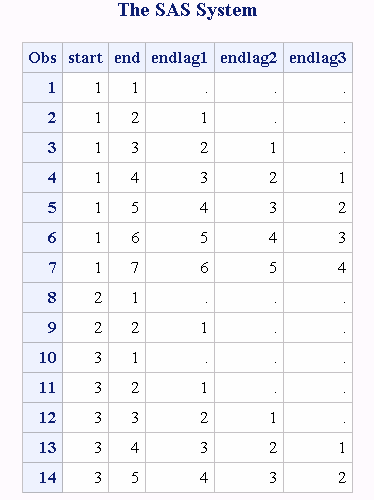### Example 3: Computing the Moving Average of a Variable

The following is an example that computes the moving average of a variable in a data set.
```/* Title: Compute the moving average of a variable
Goal: Compute the moving average of a variable through the entire data set,
of the last n observations and of the last n observations within a
BY-group.
Input:
*/
data x;
do x=1 to 10;
output;
end;
run;
/* Compute the moving average of the entire data set. */
data avg;
retain s 0;
set x;
s=s+x;
a=s/_n_;
run;
proc print;
run;
/* Compute the moving average of the last 5 observations. */
%let n = 5;
data avg (drop=s);
retain s;
set x;
s = sum (s, x, -lag&n(x)) ;
a = s / min(_n_, &n);
run;
proc print;
run;
/* Compute the moving average within a BY-group of last n observations.
For the first n-1 observations within the BY-group, the moving average
is set to missing. */
data ds1;
do patient='A','B','C';
do month=1 to 7;
num=int(ranuni(0)*10);
output;
end;
end;
run;
proc sort;
by patient;
%let n = 4;
data ds2;
set ds1;
by patient;
retain num_sum 0;
if first.patient then do;
count=0;
num_sum=0;
end;
count+1;
last&n=lag&n(num);
if count gt &n then num_sum=sum(num_sum,num,-last&n);
else num_sum=sum(num_sum,num);
if count ge &n then mov_aver=num_sum/&n;
else mov_aver=.;
run;
proc print;
run;```
Output from Computing the Moving Average of a Variable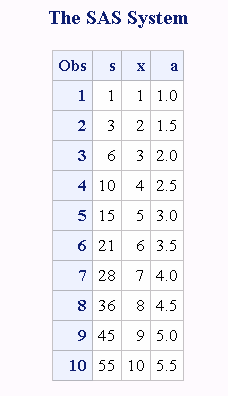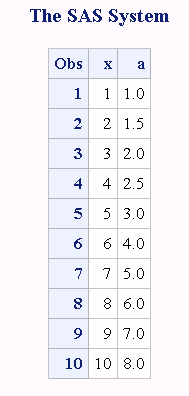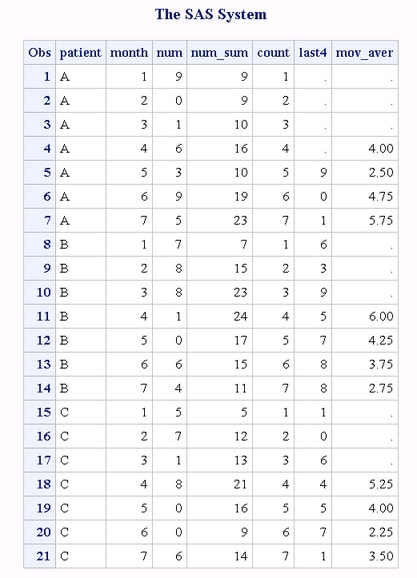### Example 4: Generating a Fibonacci Sequence of Numbers

The following example generates a Fibonacci sequence of numbers. You start with 0 and 1, and then add the two previous Fibonacci numbers to generate the next Fibonacci number.
```data _null_;
put 'Fibonacci Sequence';
n=1;
f=1;
put n= f=;
do n=2 to 10;
f=sum(f,lag(f));
put n= f=;
end;
run;```
SAS writes the following output to the log:
```Fibonacci Sequence
n=1 f=1
n=2 f=1
n=3 f=2
n=4 f=3
n=5 f=5
n=6 f=8
n=7 f=13
n=8 f=21
n=9 f=34
n=10 f=55```

### Example 5: Using Expressions for the LAG Function Argument

The following program uses an expression for the value of argument and creates a data set that contains the values for X, Y, and Z. LAG dequeues the previous values of the expression and enqueues the current value.
```data one;
input X @@;
Y=lag1(x+10);
Z=lag2(x);
datalines;
1 2 3 4 5 6
;
proc print;
title 'Lag Output: Using an Expression';
run;```
Output from the LAG Function: Using an Expression